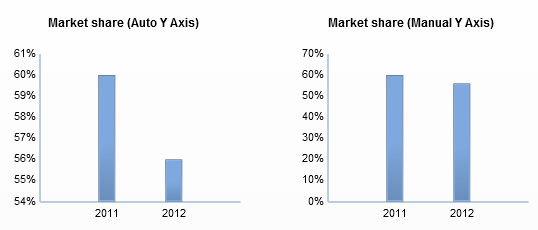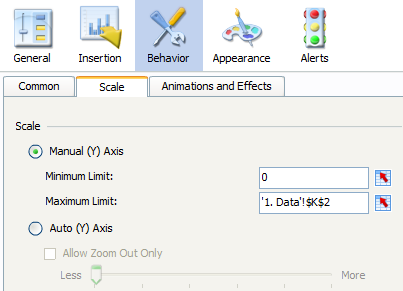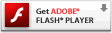# SAP BusinessObjects Dashboards: Variable maximum limits in charts

Posted by Xavier Hacking

If you use a chart in SAP BusinessObjects Dashboards (Xcelsius) you have the option to adjust the scale of the y-axis. By default Dashboards uses the automatic axis and makes up its own minimum and maximum limits. The biggest drawback of this feature is that it won’t let the y-axis start at zero all the time; this depends on the data set the chart is displaying. To make honest comparisons of data I believe a chart should show the complete picture and start its value axis at zero.

The example below shows the same numbers in two bar charts with different limits on the y-axis. Do you see what is happening here? The left chart might give you the impression of a giant decline in market share; losing more than half it. In reality, market share is down from 60% to 56%, which is a decline of only 6,67%.In Dashboards I always use the option to manually set the limits of the y-axis. But, what if you don’t know what value ranges have to be shown in the dashboard? And what if want to display multiple different sets of data in the same chart? Or you have created an option in your dashboard to filter the data and want to show some drilled subsets of the data? If you set the maximum limit too low there is a risk some values won’t be shown on the chart. If you set the maximum limit too high a data set with smaller numbers won’t show any differences between the values anymore.So we have to make the maximum limit variable to tackle this problem. We are going to use some MS Excel formulas to do this. Make sure you check the example at the end of this post.

Let’s say we’ve got a data set with the monthly results for some products. First we set up the chart and the data insertion with a selector component so we can choose which product should be shown.

Next we have to calculate the maximum value of the row of data with the MAX function: =MAX(number1, number2…).

But, we actually want to use nice rounded numbers as our maximum limit. There is an Excel formula called CEILING to do this: =CEILING(number, significance). The significance part is interesting. If we don’t put something in there the number will be rounded up to the nearest integer (10.3 turns into 11). That’s nice, but we actually want to round up to tens, hundreds, thousands ands so on. That’s where the significance part comes into play. For example, to round up to hundreds you should put 100 here, so 35 gets rounded up to 100 and 102 gets rounded up to 200.

How can we determine which significance value we should use? We are going to use some good old exponentiation for this: 102 = 100 (10 to the power 2), 103  = 1000 (10 to the power 3) and so on. If the maximum value of a row of data is 535585, we want the maximum limit to be 600000. The significance value should be 100000 in that case, which is 105 (10 to the power 5). The number of charactersin 535585 is 6. But, if we use this 6 as the power we would get 1000000 as the nearest round up value. This is 10 times too big. So we need to subtract 1 from the power (10(6-1)) to get the significance value we want!

To count the number of characters we can use the LEN function: =LEN(number). To calculate the significance value this Excel formula can be used: =10^(NumberOfCharacters-1).

To finish things of we can even make the number of divisions variable now. Just divide the maximum limit through the significance. This prevents us from getting a ‘difficult’ set of axis labels. For example, if we always show 5 divisions, and our maximum value is 90, we would get 18, 36, 54, 72, 90 as labels.

Check the example below to see what happens when selecting the several rows of data.[kml_flashembed publishmethod=”static” fversion=”8.0.0″ movie=”http://hackingsap.com/blog/wp-content/uploads/Max_Limit_example.swf” width=”650″ height=”500″ targetclass=”flashmovie”][/kml_flashembed]

HackingSAP.com - Sep 24, 2012 | Knowledge sharing, SAP BusinessObjects Dashboards, Xcelsius
Tagged | , ,

1.##### Josh Tapley
September 26, 2012

I often use something like =Round(Max(A1:A10)/5,0)*5 to round to say the nearest 5, but I like how your solution scales to the order of magnitude without adding extra logic!

– Josh

2.##### Frodo Jansen
September 28, 2012

Nice job! A couple of weeks ago I played around with the same type of formula’s as well (I wanted two graphs to have the same y-as in order to support easy comparison). I most of the time got the desired result but not all of the time. I have to check your approach with using exponentiation.

I also used a formula to determine whether 3 or 4 divisions would be best ( I consider 5 to be the max for most occasions).

3.##### Frodo Jansen
October 1, 2012

There are still some limitations to this approach that prevent it from being a generic, will-always-work, solution.

My solution isn’t perfect either so maybe we can have a look at it together.

1) Using LEN(max value) will not get you the required number if you work with decimals (e.g. the maximum value is \$1.2 million). I used =LEN(ROUNDDOWN(max value;0))
2) It doesn’t yet work for negative values. Can be solved by calculating the minimum value as well.
3) It doesn’t work well for values < 1 (often a significant smaller than 10 is needed)
4) The maximum value often equals the maximum limit. I believe it's better to have some space between the maximum value and the maximum limit.
5) There's really a big variance between the numbers of divisions. I believe a graph is most effective (looks best?) if it has 3,4 or 5 divisions. More divisions will reduce the readability of the graph.

Manually it's easy to create the perfect graph with the right number of divisions and the maximum values but it quit difficult to automate.

Regards,
Frodo

•##### Xavier Hacking
October 17, 2012

Excellent comment! Thanks!

4.##### Alasdair
December 4, 2015

Hi Xavier – this is exactly what I need. The example no longer appears. Can you point me to it?

Thanks,
Alasdair

•